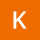Home
IT Knowledge
Inspiration
Languages
EN

# Python - math.log() method example

0 points
Created by:Keisha-Acosta
260

The `math.log()` method returns the natural logarithm (base e) of a number.

``````import math

print(math.log(1))      # 0
print(math.log(7))      # 1.9459101490553132
print(math.log(10))     # 2.3025850929940460
print(math.log(100))    # 4.6051701859880920
print(math.log(1000))   # 6.9077552789821370

print(math.e)  # 2.718281828459045

# Logarithm with custom base is placed in the below example.``````

The `math.log()` method is presented on the following chart:

## 1. Documentation

 Syntax ``math.log(x, newBase)`` Parameters `x`- value in the range `0` to `infinitive`. `newBase` - logarithm base. Result `number` value calculated as ln(x) mathematical function. If `x` is negative it returns ValueError. If `x` is equal to `0` it returns ValueError. If `x` is equal to `infinity` it returns `infinity`. Description `log` is a method that takes one parameter and returns an approximation of the ln(x) function (natural logarithm function - logarithm with base `e`).

## 2. Logarithm with custom base example

This example shows a logarithmic function calculation with its own base.

``````import math

def calculate_logarithm(log_base, x):
a = math.log(x)
b = math.log(log_base)
return a / b

# Logarithm with custom base:
#                          base     x              y
print(calculate_logarithm( 2,       2       ) )  # 1.0
print(calculate_logarithm( 2,       4       ) )  # 2.0
print(calculate_logarithm( math.e,  math.e  ) )  # 1.0
print(calculate_logarithm( 3,       9       ) )  # 2.0
print(calculate_logarithm( 3,       81      ) )  # 4.0
print(calculate_logarithm( 10,      10      ) )  # 1.0``````

## References

1. Natural logarithm - Wikipedia